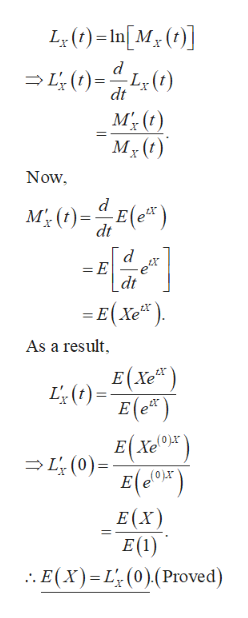# 6. Consider the function Lx (t) = In (Mx (t)). Show that E(X) = L* (0) and Var(X) = L% (0).

Question
108 views

check_circle

Step 1

Introduction:

Here, MX (t) denotes the moment generating function (mgf) of the random variable X; thus, MX (t) = E (etX).

The quantity LX (t) is the natural logarithm of MX (t).

Remember that MX (t), LX (t) are functions of t, and not that of x. Thus, all the derivatives are done here with respect to t, and not with respect to x.

Step 2

Calculation to prove E (X) = L՜ (0):

First, calculate the first order derivative of LX (t) with respect to t. After obtaining the d...help_outlineImage TranscriptioncloseL()-In[M (] L(t)L(t) dt MT, (t) Now M0)E) dt = E e dt = E(Xe) As a result E(Xe) L() E (e) EXe L(0) E(XT) E(X) E(1) . E(x) L (0)(Proved) fullscreen

### Want to see the full answer?

See Solution

#### Want to see this answer and more?

Solutions are written by subject experts who are available 24/7. Questions are typically answered within 1 hour.*

See Solution
*Response times may vary by subject and question.
Tagged in

### Statistics